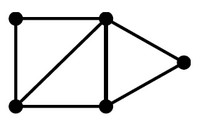# Graph - (Edge | Links | Arcs | Lines | Arrows) - Association

An edge model a relationship between two node in a graph. Every edge model therefore a binary relationship (relationship between two elements) .

An Edge is also known as:

A edge in a property graph always has:

• a direction, (that can be navigated regardless of direction)
• a type,
• two nodes (a start node and an end node if directed).
• quantitative properties (such as weights, costs, distances, ratings, time intervals, or strengths)

An existing edge will never point to a non-existing node.

A sequence of edges between two nodes is called a path

## Representation

Edge can be represented in term of linear algebra vectors

Recommended Pages(Network|Graph) - Directed Graph (or digraph)

A directed graph (or digraph) is a graph, where the edges have a direction associated with them. Directed edges are suitable for modeling asymmetric relations undirected Data flow modeling ...Automata - Transition

In a automata, a Transition is the fact of going from one state to another and is represent by a edge in a graph. It's a function that: takes as arguments a state and an input symbol (The transition...Automaton (State Machine)

Automaton (State Machine) Automata theory is the study of abstract computing devices or machines. The word automata (the plural of automaton) comes from the Greek word αὐτόματα, which means...Data Processing - Arc

The arcs shows the data dependencies among operators Arcs are edge in a graph data flow.Graph (Network - Nodes and edges)

A graph is a set of vertices connected by edges. See Data representation that naturally captures complex relationships is a graph (or network). Except of the special graph that a tree is, the data...Graph - (Node | Vertice | Vertex | Point)

A node in a graph model an entity The model graph contains connected the nodes: containing any number of attributes (key-value-pairs). tagged with labels (to label, attach metadata—​index or...Graph - (Node) Attribute

Node Attributes are descriptive attribute of a node or edge in a graph Egde attribute: weights, costs, distances, ratings, time intervals, or strengthsadjacent is a graph operator that tests if there is an edge between two vertices; The two vertices have the following binary relation, if the test is: true: is adjacent to false: is not adjacent...Graph - Data Structure (Physical Representation)

A graph is represented generally in a physical data structure The graph is composed of two set. a set of vertices (node) Node a b c and set of egde represented for a : directed graph...Graph - Degree

In a graph, the degree of a vertex (node) is the number of edges that touch the node (vertex) (ie the number of adjacent edges) degree is also known as valency The original 'six degrees of separation'...